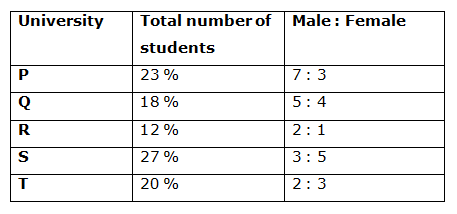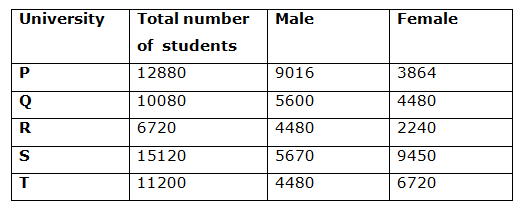# CWC/FCI Prelims 2019 – Quantitative Aptitude Questions (Day-28)

Dear Readers, Exam Race for the Year 2019 has already started, To enrich your preparation here we are providing new series of Practice Questions on Quantitative Aptitude – Section for CWC/FCI Exam. Aspirants, practice these questions on a regular basis to improve your score in aptitude section. Start your effective preparation from the right beginning to get success in upcoming CWC/FCI Exam.

[WpProQuiz 5732]

Directions (1 – 5): In each of the following questions, two equations (a) and (b) are given. You have to solve them and find the correct option.

a) x > y

b) x < y

c) x ≤ y

d) x ≥ y

e) x = y Or cannot be determined

1)

a) 5x2-13x-6=0

b) 6y2+29y+35=0

2)

a)12x-14y+38=0

b) 4x+7y-209=0

3)

a) x2+28y+195=0

b) y2-4y-221=0

4)

a) 20x2+27x+9=0

b) 2y2-39y+54=0

5)

a) 7x+8y=42

b) 3x+5y=18

Directions (6 – 10): Study the following information carefully and answer the given questions:

Following table shows the percentage distribution of total number of students who completed their graduation from different universities and the ratio of male to female among them.

Total number of students = 560006) Find the total number of male graduates from the given universities together?

a) 29246

b) 25782

c) 33567

d) 37895

e) None of these

7) Total number of female graduates from university Q and R is approximately what percentage of total number of male graduates from university P and T?

a) 32 %

b) 44 %

c) 68 %

d) 50 %

e) 36 %

8) Find the ratio between the total number of male graduates from university Q, R and T together to that of female graduates from university P, R and S together?

a) 8576 : 7115

b) 9254 : 8471

c) 7280 : 7777

d) 5890 : 4783

e) None of these

9) Total number of graduates from university P and Q together is approximately what percentage more/less than the total number of graduates from university R and S together?

a) 8 % less

b) 5 % more

c) 12 % more

d) 15 % less

e) 18 % more

10) In which university, the highest number of female graduates completed their graduation?

a) University P

b) University Q

c) University R

d) University S

e) University T

Direction (1-5) :

a) 5x2-13x-6=0

5x2-15x+2x-6=0

(5x+2)(x-3)=0

x=-2/5 or 3

b) 6y2+29y+35=0

6y2+15y+14y+35=0

y=-5/2 or -7/3

Hence, x>y

12x -14y+38=0 ……….. (1)

4x+7y-209=0 ……………… (2)

Multiplying (2) by 2 and adding to (1), we have

x= 19 and y= 19

Thus, x= y

a) x2+28y+195=0

(x+13)(x+15)=0

x=-13 or -15

b) y2-4y-221=0

(y-17)(y+13)=0

y=-13 or 17

Hence, x ≤ y

a) 20x2+27x+9=0

20x2+15x+12x +9=0

x=-3/5 or -3/4

b) 2y2-39y+54=0

2y2-36y-3y+54=0

(2y-3)(y-18)=0

y= 1.5 and 18

Hence x<y

7x+8y=42 ………….. (1)

3x+5y=18 ………….. (2)

Multiplying (1) by 3 and (2) by 7, and after solving them we have

x= 6 and y=0

Thus, x>y

Directions (6 – 10):The total number of male graduates from the given universities together

= > 9016 + 5600 + 4480 + 5670 + 4480

= > 29246

Total number of female graduates from university Q and R

= > 4480 + 2240 = 6720

Total number of male graduates from university P and T

= > 9016 + 4480 = 13496

Required % = (6720/13496)*100 = 49.79 % = 50 %

The total number of male graduates from university Q, R and T together

= > 5600 + 4480 + 4480 = 14560

The total number of female graduates from university P, R and S together

= > 3864 + 2240 + 9450 = 15554

Required ratio = 14560: 15554 = 7280: 7777

Required % = {[(23+ 18) – (12 + 27)]/(12 + 27)}*100

= > {(41 – 39)/39}*100 = 5.128 % = 5 % more